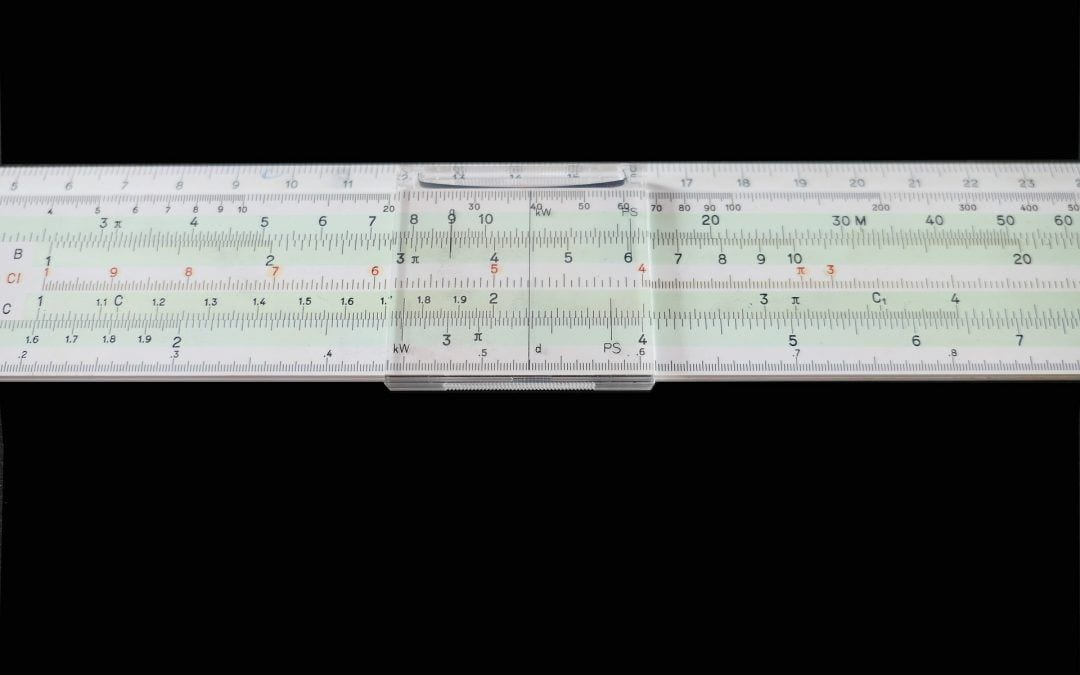Select PageHow to use the COLUMNS formula in Google Sheets

Let us say we have a range of cells in a spreadsheet, and we want to know how many columns it has spread across. If there are only a few of them, we could probably do a quick observation and manually count the number. But there can be instances where columns in a...How to use the FLOOR formula in Google Sheets

While there are infinite rational numbers in mathematics, we don’t usually deal with all of them. That’s because, in real life, it isn’t even feasible. Consider currency for instance. We may make many money transaction calculations – interest, exchange rates, etc. For...How to use the LOG formula in Google Sheets

In this post, we learned the behavior of natural logarithm and the usage of LN formula. While we can agree that the logarithm evaluated upon base e is quite useful in many fields, it isn’t as flexible. For instance, we can not evaluate the logarithm for a base that is...How to use the ROUND formula in Google Sheets

While working with spreadsheets, we tend to come across many numbers that are rational. Some of them have more decimal values after the decimal point than desired. And our requirement may be such that we need to confine the rational values to the desired number of...How to use the COUNTUNIQUE Google Sheets formula

While working with spreadsheets, we are bound to do many sorts of calculations. And we will, at times need to calculate unique occurrences of a dataset. The number of unique customers that shopped at a store, for example. How do we achieve this easily? We can, with...How to use the LARGE formula in Google Sheets

Number crunching is one of the primary activities that the spreadsheets applications are built for. We all know how to find out the maximum value from a dataset, using the MAX formula. What if we need to find out the second largest value from the set of numeric...How to use the COVAR formula in Google Sheets

Analyzing the data leads us to multiple interpretations as to what the underlying nature is. And we have many important statistical tools and metrics that help us with this exercise. One such important metric that mathematicians have at their disposal is the...How to use the CORREL formula in Google Sheets

Analyzing data entails going through various statistical measures to understand the underlying nature and trends. Probably, one of the most commonly used statistical methods is Correlation. We use it to gauge the extent of relationship, dependence or closeness of two...How to use the NORMSDIST formula in Google Sheets

In this post, learn how to calculate the normal cumulative distribution by using the NORMSDIST formula in Google Sheets. The normal cumulative distribution is one of the most widely used probability distributions in statistics. We can derive a specialized case of...How to use the NORMSINV formula in Google Sheets

We are aware of the important role, the normal cumulative distribution plays in the field of statistics. We can derive a specialized case of normal cumulative distribution when mean is zero and the variance is 1. This is called the standard normal cumulative...How to use the STDEV formula in Google Sheets

We carry out most of our data analysis tasks on spreadsheets applications. And we will need to arrive at some key statistical metrics to understand the nature of the dataset. Standard deviation is one such metric that helps us quantify the amount of variation or...How to use the VAR formula in Google Sheets

Data is what the spreadsheets are built for – and helping us analyze it is its primary goal. There are some key metrics one needs to use while statistically analyzing the datasets. And variance is an important one of them. It measures how far a set of numbers are...

Questions or remarks?

If you have any questions or remarks on this post, please visit our community and do not hesitate to contact us there! Join

What is Sheetgo?

Sheetgo is a cloud-based software that allows you to create and automate workflows straight from your spreadsheet. Try itDon't hesitate to reach us anytime! support@sheetgo.com

Resources

© 2016-2019 SHEETGO EUROPE SL